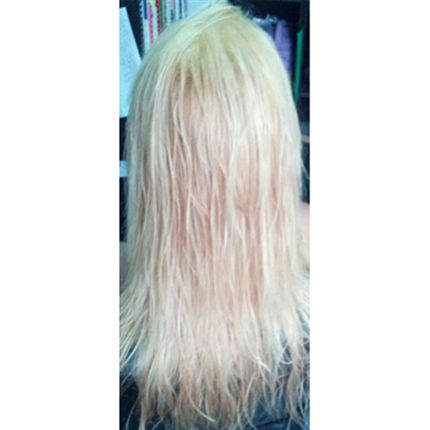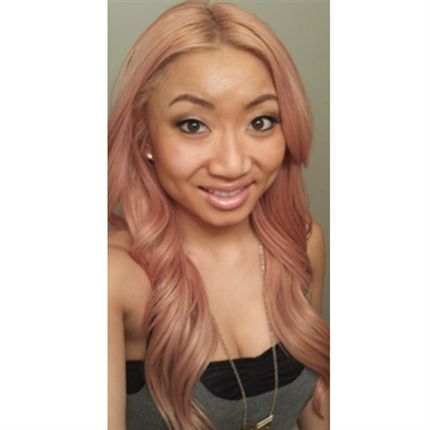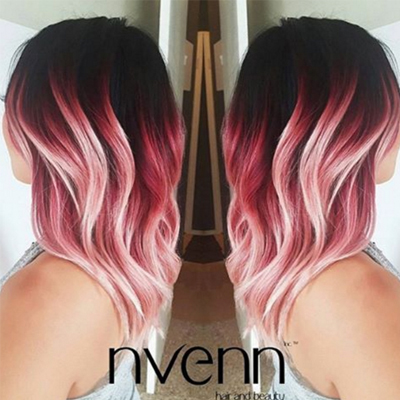Transforming Dark Red to OMG Ombré!
May 30, 2016

# Transforming Dark Red to OMG Ombré!

VIEW ALL PHOTOS VIEW SLIDESHOW
GET THE HOW-TO• Natural/Starting Level:

Previously colored with CHI Ionic Permanent Shine in 5RV (Medium Red Violet)

• Formula A:

BLONDME Premium Lift 9+ + 20-volume developer

• Formula B (highlights):

BLONDME Premium Lift 9+ + 7-volume developer

• Formula C:

Equal parts IGORA ROYAL 7.0 + 7.1

• Formula D:

IGORA ROYAL 7.0

• Formula E:

Equal parts IGORA ROYAL 8.11 + 6.12

• Formula F:

IGORA ROYAL 9.1 + 9.5-22

• Formula G (toner):

Equal parts IGORA ROYAL 9.5-1 + 9.5-22 + 10-volume developer

• Formula H (ombré ends):

IGORA ROYAL 9.5-18 Rose + 10-volume developer

• Formula I (ombré roots):

Vibrance 1N

• Formula J (ombré midlengths color melt):

IGORA ROYAL 9.98 + 9.5-18# Transforming Dark Red to OMG Ombré!

We love a good color journey, especially when the destination is as stunning as this dynamic coral ombré. This hand-painted masterpiece comes to us from nvenn hair and beauty bar in Edmonton and Calgary, AB, Canada. The team at nvenn spent 12 months and five sessions taking their client from a deep wine-red to a coral balayage blend, and below, they walk us through each stage of the process.

“Our number one priority in color corrections is to maintain the integrity of the hair as we move toward our client’s hair goals,” say Jessica Lee and Veronica Lee, co-founders of nvenn. “Depending on the job, this may take a few sittings for their hair to be exactly where they want it to be. Our promise, though, is that they do not leave with ‘ugly’ hair that looks like it is in the middle of a color correction.”

Want to be featured? Tag your pics to #behindthechair!

## COLOR FORMULAS• Natural/Starting Level:

Previously colored with CHI Ionic Permanent Shine in 5RV (Medium Red Violet)

• Formula A:

BLONDME Premium Lift 9+ + 20-volume developer

• Formula B (highlights):

BLONDME Premium Lift 9+ + 7-volume developer

• Formula C:

Equal parts IGORA ROYAL 7.0 + 7.1

• Formula D:

IGORA ROYAL 7.0

• Formula E:

Equal parts IGORA ROYAL 8.11 + 6.12

• Formula F:

IGORA ROYAL 9.1 + 9.5-22

• Formula G (toner):

Equal parts IGORA ROYAL 9.5-1 + 9.5-22 + 10-volume developer

• Formula H (ombré ends):

IGORA ROYAL 9.5-18 Rose + 10-volume developer

• Formula I (ombré roots):

Vibrance 1N

• Formula J (ombré midlengths color melt):

IGORA ROYAL 9.98 + 9.5-18

## HOW-TO STEPS•• Natural/Starting Level:

Previously colored with CHI Ionic Permanent Shine in 5RV (Medium Red Violet)

• Formula A:

BLONDME Premium Lift 9+ + 20-volume developer

• Formula B (highlights):

BLONDME Premium Lift 9+ + 7-volume developer

• Formula C:

Equal parts IGORA ROYAL 7.0 + 7.1

• Formula D:

IGORA ROYAL 7.0

• Formula E:

Equal parts IGORA ROYAL 8.11 + 6.12

• Formula F:

IGORA ROYAL 9.1 + 9.5-22

• Formula G (toner):

Equal parts IGORA ROYAL 9.5-1 + 9.5-22 + 10-volume developer

• Formula H (ombré ends):

IGORA ROYAL 9.5-18 Rose + 10-volume developer

• Formula I (ombré roots):

Vibrance 1N

• Formula J (ombré midlengths color melt):

IGORA ROYAL 9.98 + 9.5-18

1

During her first session, apply Formula A from the roots to the ends.

After visit 1. Total price = \$115+.

•• Natural/Starting Level:

Previously colored with CHI Ionic Permanent Shine in 5RV (Medium Red Violet)

• Formula A:

BLONDME Premium Lift 9+ + 20-volume developer

• Formula B (highlights):

BLONDME Premium Lift 9+ + 7-volume developer

• Formula C:

Equal parts IGORA ROYAL 7.0 + 7.1

• Formula D:

IGORA ROYAL 7.0

• Formula E:

Equal parts IGORA ROYAL 8.11 + 6.12

• Formula F:

IGORA ROYAL 9.1 + 9.5-22

• Formula G (toner):

Equal parts IGORA ROYAL 9.5-1 + 9.5-22 + 10-volume developer

• Formula H (ombré ends):

IGORA ROYAL 9.5-18 Rose + 10-volume developer

• Formula I (ombré roots):

Vibrance 1N

• Formula J (ombré midlengths color melt):

IGORA ROYAL 9.98 + 9.5-18

2

At her second session, apply Formula A from the roots to the ends again.
After visit 2. Total price = \$115+.

•• Natural/Starting Level:

Previously colored with CHI Ionic Permanent Shine in 5RV (Medium Red Violet)

• Formula A:

BLONDME Premium Lift 9+ + 20-volume developer

• Formula B (highlights):

BLONDME Premium Lift 9+ + 7-volume developer

• Formula C:

Equal parts IGORA ROYAL 7.0 + 7.1

• Formula D:

IGORA ROYAL 7.0

• Formula E:

Equal parts IGORA ROYAL 8.11 + 6.12

• Formula F:

IGORA ROYAL 9.1 + 9.5-22

• Formula G (toner):

Equal parts IGORA ROYAL 9.5-1 + 9.5-22 + 10-volume developer

• Formula H (ombré ends):

IGORA ROYAL 9.5-18 Rose + 10-volume developer

• Formula I (ombré roots):

Vibrance 1N

• Formula J (ombré midlengths color melt):

IGORA ROYAL 9.98 + 9.5-18

3

During her third session, highlight the entire head using Formula B, applying color between the foils. Apply Formula C to the first inch of new growth, Formula D to the midshaft and Formula E to the previously-lightened ends.

•• Natural/Starting Level:

Previously colored with CHI Ionic Permanent Shine in 5RV (Medium Red Violet)

• Formula A:

BLONDME Premium Lift 9+ + 20-volume developer

• Formula B (highlights):

BLONDME Premium Lift 9+ + 7-volume developer

• Formula C:

Equal parts IGORA ROYAL 7.0 + 7.1

• Formula D:

IGORA ROYAL 7.0

• Formula E:

Equal parts IGORA ROYAL 8.11 + 6.12

• Formula F:

IGORA ROYAL 9.1 + 9.5-22

• Formula G (toner):

Equal parts IGORA ROYAL 9.5-1 + 9.5-22 + 10-volume developer

• Formula H (ombré ends):

IGORA ROYAL 9.5-18 Rose + 10-volume developer

• Formula I (ombré roots):

Vibrance 1N

• Formula J (ombré midlengths color melt):

IGORA ROYAL 9.98 + 9.5-18

4

Tone her highlights using Formula F.
After visit 3. Total price = \$265+.

•• Natural/Starting Level:

Previously colored with CHI Ionic Permanent Shine in 5RV (Medium Red Violet)

• Formula A:

BLONDME Premium Lift 9+ + 20-volume developer

• Formula B (highlights):

BLONDME Premium Lift 9+ + 7-volume developer

• Formula C:

Equal parts IGORA ROYAL 7.0 + 7.1

• Formula D:

IGORA ROYAL 7.0

• Formula E:

Equal parts IGORA ROYAL 8.11 + 6.12

• Formula F:

IGORA ROYAL 9.1 + 9.5-22

• Formula G (toner):

Equal parts IGORA ROYAL 9.5-1 + 9.5-22 + 10-volume developer

• Formula H (ombré ends):

IGORA ROYAL 9.5-18 Rose + 10-volume developer

• Formula I (ombré roots):

Vibrance 1N

• Formula J (ombré midlengths color melt):

IGORA ROYAL 9.98 + 9.5-18

5

At her fourth session, apply Formula A from the roots to the ends again.

• 6

Apply Formula G from roots to ends.

•• Natural/Starting Level:

Previously colored with CHI Ionic Permanent Shine in 5RV (Medium Red Violet)

• Formula A:

BLONDME Premium Lift 9+ + 20-volume developer

• Formula B (highlights):

BLONDME Premium Lift 9+ + 7-volume developer

• Formula C:

Equal parts IGORA ROYAL 7.0 + 7.1

• Formula D:

IGORA ROYAL 7.0

• Formula E:

Equal parts IGORA ROYAL 8.11 + 6.12

• Formula F:

IGORA ROYAL 9.1 + 9.5-22

• Formula G (toner):

Equal parts IGORA ROYAL 9.5-1 + 9.5-22 + 10-volume developer

• Formula H (ombré ends):

IGORA ROYAL 9.5-18 Rose + 10-volume developer

• Formula I (ombré roots):

Vibrance 1N

• Formula J (ombré midlengths color melt):

IGORA ROYAL 9.98 + 9.5-18

7

Apply Formula H from roots to ends.
After visit 4. Total price = \$255+.

•• Natural/Starting Level:

Previously colored with CHI Ionic Permanent Shine in 5RV (Medium Red Violet)

• Formula A:

BLONDME Premium Lift 9+ + 20-volume developer

• Formula B (highlights):

BLONDME Premium Lift 9+ + 7-volume developer

• Formula C:

Equal parts IGORA ROYAL 7.0 + 7.1

• Formula D:

IGORA ROYAL 7.0

• Formula E:

Equal parts IGORA ROYAL 8.11 + 6.12

• Formula F:

IGORA ROYAL 9.1 + 9.5-22

• Formula G (toner):

Equal parts IGORA ROYAL 9.5-1 + 9.5-22 + 10-volume developer

• Formula H (ombré ends):

IGORA ROYAL 9.5-18 Rose + 10-volume developer

• Formula I (ombré roots):

Vibrance 1N

• Formula J (ombré midlengths color melt):

IGORA ROYAL 9.98 + 9.5-18

8

Color-melt the hair to create an ombré look from black to magenta to pastel pink using Formulas I, J and H.
Finished result (after visit 5). Total price = \$95+.

[class^="wpforms-"]
[class^="wpforms-"]
[class^="wpforms-"]
[class^="wpforms-"]